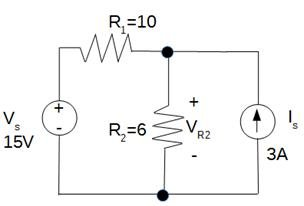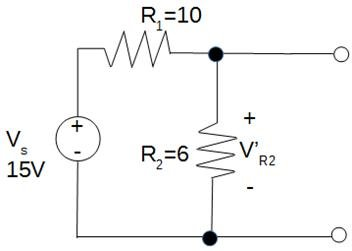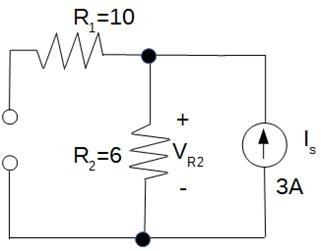# Electrical Engineering Assignment Example

Problem 1. Find the voltage VR2 in the circuit on the Figure 1 below.Figure 1. Circuit for Problem 1

Solution

The problem 1 can be solved using the superposition method. This method is based on the elimination of all sources except one at a time, utilizing a series/parallel analysis in order to determine drops of voltage in the modified network for each source of the power.

The right source can be activating at the first step.Now the left source will be set on zero (see the picture below):Now it could be seen that R1 became parallel to R2.

The total value of VR2: